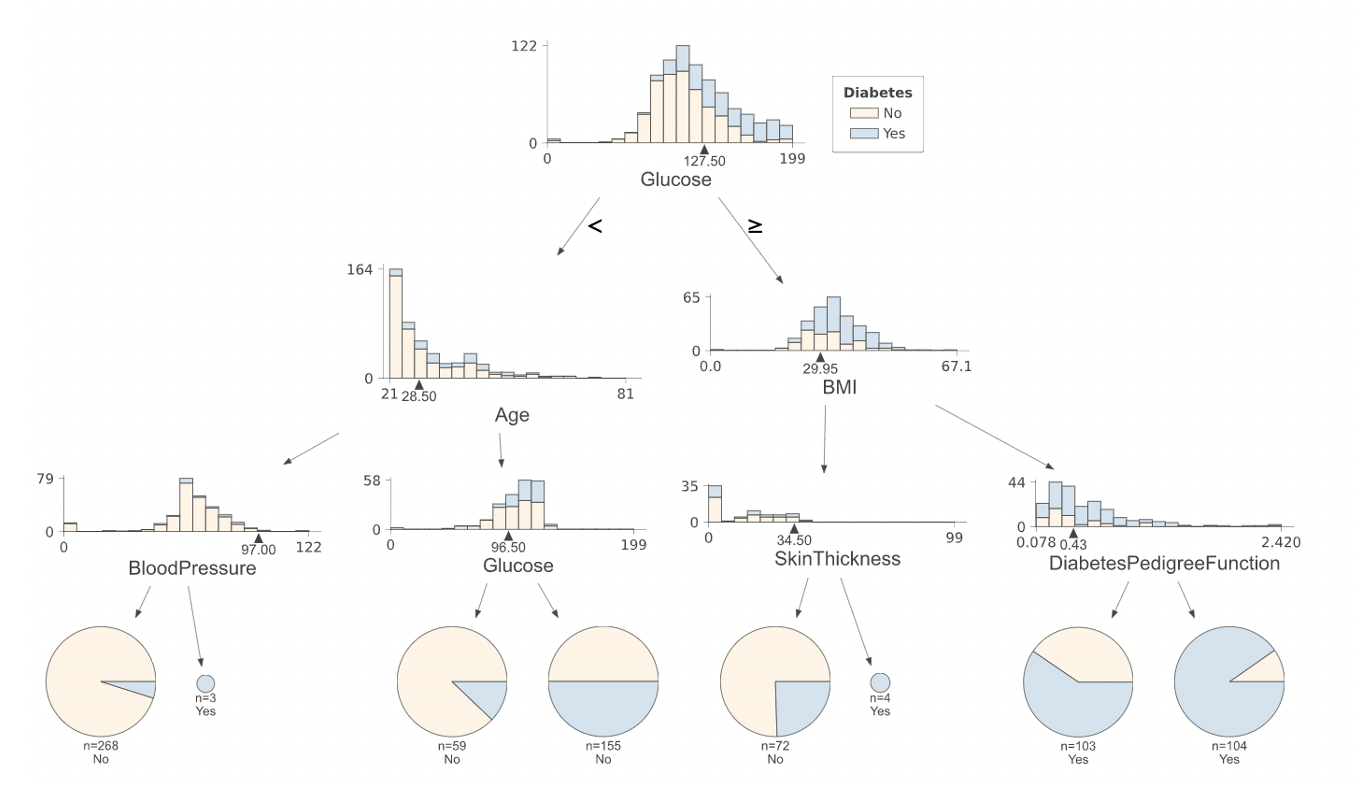# Title¶

Exercise 1: Bagging vs Random Forest (Tree correlation)

# Description¶

## How does Random Forest improve on Bagging?¶

The goal of this exercise is to investigate the correlation between randomly selected trees from Bagging and Random Forest.

# Instructions:¶

• Read the dataset diabetes.csv as a pandas dataframe, and take a quick look at the data.
• Split the data into train and validation sets.
• Define a BaggingClassifier model that uses DecisionTreClassifier as its base estimator.
• Specify the number of bootstraps as 1000 and a maximum depth of 3.
• Fit the BaggingClassifier model on the train data.
• Use the helper code to predict using the mean model and individual estimators. The plot will look similar to the one given below.
• Predict on the test data using the first estimator and the mean model.
• Compute and display the validation accuracy
• Repeat the modeling and classification process above, this time using a RandomForestClassifier.

# Your final output should look something like this:¶# Hints:¶

sklearn.train_test_split() : Split arrays or matrices into random train and test subsets.

sklearn.ensemble.BaggingClassifier() : Returns a Bagging classifier instance.

sklearn.tree.DecisionTreeClassifier() : A Tree classifier can be used as the base model for the Bagging classifier.

sklearn.ensemble.andomForestClassifier() : Defines a Random forest classifier.

sklearn.metrics.accuracy_score(y_true, y_pred) : Accuracy classification score.

In :
#!pip install -qq dtreeviz
import os, sys
sys.path.append(f"{os.getcwd()}/../")

In :
# Import the main packages

import numpy as np
import pandas as pd
import matplotlib.pyplot as plt
from sklearn.ensemble import RandomForestClassifier
from sklearn.ensemble import BaggingClassifier

from sklearn.model_selection import train_test_split
from sklearn.tree import DecisionTreeClassifier
from sklearn.model_selection import train_test_split
from sklearn.metrics import accuracy_score
from dtreeviz.trees import dtreeviz

%matplotlib inline

colors = [None,  # 0 classes
None,  # 1 class
['#FFF4E5','#D2E3EF'],# 2 classes
]

from IPython.display import Markdown, display
def printmd(string):
display(Markdown(string))

In :
# Read the dataset and take a quick look


In :
### edTest(test_assign) ###
# Assign the predictor and response variables.
# "Outcome" is the response and all the other columns are the predictors

X = __
y = __

In :
# Fix a random_state and split the data
# into train and validation sets

random_state = 144

X_train, X_val, y_train,y_val = train_test_split(__,__,
train_size = 0.8,
random_state =random_state)


## Bagging Implementation¶

In :
# Define a Bagging classifier with randon_state as above
# and with a DecisionClassifier as a basemodel
# We fix the max_depth variable to 20 for all trees
max_depth = 20

# Set the maximum depth to be max_depth and use 100 estimators
n_estimators = 1000
basemodel = __(max_depth=__,
random_state=__)

bagging = BaggingClassifier(base_estimator=basemodel,
n_estimators=n_estimators)
# Fit the model on the training set

bagging.fit(__, __)

In :
### edTest(test_bagging) ###
# We make predictions on the validation set

predictions = bagging.predict(X_val)

# compute the accuracy on the validation set

acc_bag = round(accuracy_score(predictions, y_val),2)

print(f'For Bagging, the accuracy on the validation set is {acc_bag}')


## Random Forest implementation¶

In :
# Define a Random Forest classifier with randon_state as above

# Set the maximum depth to be max_depth and use 100 estimators

random_forest = __(max_depth=max_depth,
random_state=random_state,
n_estimators=n_estimators)

# Fit the model on the training set
random_forest.fit(__, __)

In :
### edTest(test_RF) ###
# We make predictions on the validation set

predictions = random_forest.predict(X_val)

# compute the accuracy on the validation set

acc_rf = round(accuracy_score(predictions, y_val),2)

print(f'For Random Forest, the accuracy on the validation set is {acc_rf}')


## Visualizing the trees - Bagging¶

In :
# Reducing the max_depth for visualization

max_depth = 3

basemodel = DecisionTreeClassifier(max_depth=max_depth,
random_state=random_state)

bagging = BaggingClassifier(base_estimator=basemodel,
n_estimators=1000)

# Fit the model on the training set

bagging.fit(X_train, y_train)

# Selecting two trees at random

bagvati1 = bagging.estimators_
bagvati2 = bagging.estimators_

In :
vizA = dtreeviz(bagvati1, df.iloc[:,:8],df.Outcome,
feature_names = df.columns[:8],
target_name = 'Diabetes', class_names= ['No','Yes']
,orientation = 'TD',
colors={'classes':colors},
label_fontsize=14,
ticks_fontsize=10,
)
printmd('   Bagging Tree 1  ')
vizA

In :
vizB = dtreeviz(bagvati2, df.iloc[:,:8],df.Outcome,
feature_names = df.columns[:8],
target_name = 'Diabetes', class_names= ['No','Yes']
,orientation = 'TD',
colors={'classes':colors},
label_fontsize=14,
ticks_fontsize=10,
scale=1.1
)
printmd('   Bagging Tree 2  ')
vizB


## Visualizing the trees - Random Forest¶

In :
# Reducing the max_depth for visualization

max_depth = 3

random_forest = RandomForestClassifier(max_depth=max_depth, random_state=random_state, n_estimators=1000,max_features = "sqrt")

# Fit the model on the training set

random_forest.fit(X_train, y_train)

# Selecting two trees at random

forestvati1 = random_forest.estimators_
forestvati2 = random_forest.estimators_[__]

In :
vizC = dtreeviz(forestvati1, df.iloc[:,:8],df.Outcome,
feature_names = df.columns[:8],
target_name = 'Diabetes', class_names= ['No','Yes']
,orientation = 'TD',
colors={'classes':colors},
label_fontsize=14,
ticks_fontsize=10,
scale=1.1
)
printmd('   Random Forest Tree 1  ')
vizC

In :
vizD = dtreeviz(forestvati2, df.iloc[:,:8],df.Outcome,
feature_names = df.columns[:8],
target_name = 'Diabetes', class_names= ['No','Yes']
,orientation = 'TD',
colors={'classes':colors},
label_fontsize=14,
ticks_fontsize=10,
scale=1.1
)
printmd('   Random Forest Tree 2  ')
vizD


## Mindchow 🍲¶

• Change the max_depth of Bagging and Random Forest to see different trees. Which one gives different trees?
• Change the max_features in RandomForestClassifier to 8. How is it affecting the correlation between the trees?